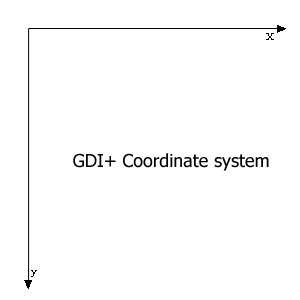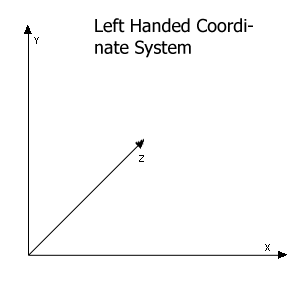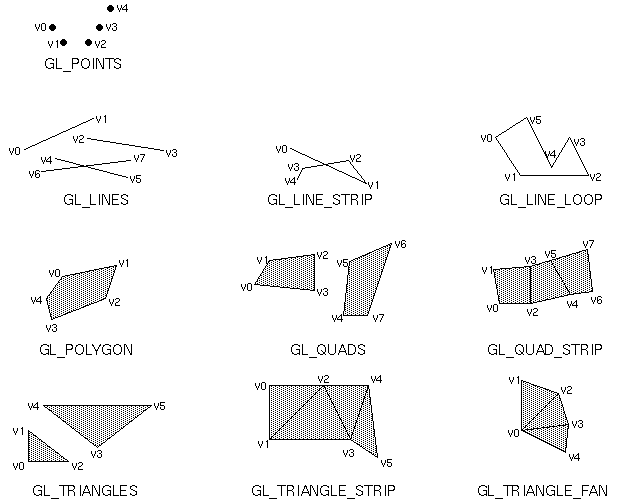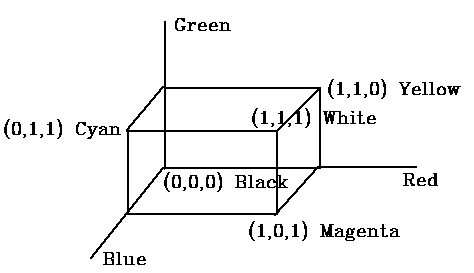In this article I want to explain couple of more things. First of all, I would like to cover the OpenGL Coordinate System.

Hi and welcome back to one more article about OpenGL. Last time we created a Windows Forms application with an OpenGLControl on it so our OpenGL could draw a box. In this article I want to explain couple of more things. First of all, I would like to cover the OpenGL Coordinate System. Of course I cant explain everything about it but this is what you will need to get the point in the following articles. Second I want to explain how we can draw stuff on the screen. In the article OpenGL using csGL I already showed you how to draw simple graphics objects.

OpenGL Coordinate System for Beginners

I wont go in much detail about the OpenGL coordinate system but I will tell you what you need to get the point in my following articles.

OpenGL doesnt use the same coordinate system as GDI+ uses. As we all know the GDI+ coordinate system has only an x and a y coordinate where x is the horizontal and y is the vertical. The value of x and y on the upper left corner is 0,0, that means the starting point of GDI+ coordinate system is upper-left corner. You probably know that OpenGL draws in 3D coordinate system, while GDI+ is a 2D coordinate system. For drawing in 3D you need at least 3 coordinates because you need to go in dept. The OpenGL coordinate system uses y x and z where 0,0,0 is the precise middle of the screen. At default youre looking at the negative z direction. That is why we used glTranslatef(0.0f, 0.0f, -6.0f) so we draw at a minus 6 coordinate to make it visible for us. The OpenGL supports another coordinate the so called w coordinate this is for dividing all the coordinates and it will be somewhat like this ( x/w, y/w, z/w ) but this isnt a very common used coordinate. So to make a 2d rectangle we have to specify 4 corners. If we want to make a rectangle with an overall length of 2 we do it like this.

glVertex3f(1.0f, -1.0f, 0.0f);
glVertex3f(1.0f, 1.0f, 0.0f);
glVertex3f(-1.0f, 1.0f, 0.0f);
glVertex3f( -1.0f, -1.0f, 0.0f);

We start at the upper left corner and we go clockwise to the upper right corner then to the botto right corner and at last the down left corner.

NOTE: that the OpenGL coordinate system is also called a left handed coordinate system because you can make the same coordinate system with your left hand try to figure it out.Please Note: Every gl command is prefix with GL. this because all functions are in CsGL.OpenGL.GL if you have Visual Studio .Net its really easy press GL. and it will show all the functions in it.

OpenGL Basics

Now we got that covered we can start with drawing in OpenGL.
As you may saw in the previous article all drawing is done between glBegin(uint mode) and glEnd(). As you saw the uint mode was GL_QUADS this is for drawing quads but there are times when you want to draw a line you will do it like this.

GL.glBegin(GL.GL_LINES);
GL.glColor3f(0.5f,0.5f,0.0f);
GL.glVertex3f(-2.0f, 2.0f, 0.0f);
GL.glVertex3f(2.0f, -2.0f, 0.0f);
GL.glEnd();

You have to put it in the base code in the glDraw function where I commented
// TODO: Draw a box or something
Now compile and run your program (F5 Vs.Net ShortCut).
What you will see is a dark green line that goes from upper left corner to bottom right corner of the window.

Now were going to try to make a 2D box. Because we dont make it too big so we use ribs of 2. It will look like this.

GL.glBegin(GL.GL_LINES);
GL.glColor3f(0.5f, 1.0f, 0.5f);
GL.glVertex3f(-1.0f, 1.0f, 0.0f);
GL.glVertex3f(1.0f, 1.0f, 0.0f);
GL.glVertex3f(1.0f, -1.0f, 0.0f);
GL.glVertex3f(-1.0f, -1.0f, 0.0f);
GL.glEnd();

Run your program once more and notice that you only see 2 lines, one from (-1.0, 1.0) to (1.0, 1.0) and the other from (1.0, -1.0) to (-1.0, -1.0).
Ok to solve this we use GL_LINE_STRIP this will loop 1 line thru all points but dont end at the beginning point for this we use GL_LINE_LOOP.
One more thing to draw only points we use GL_POINTS.
I strongly suggest to try all these to see the difference.

Before we go any further I want to show you something I got from OpenGL Redbook.

 Value Meaning GL_POINTS individual points GL_LINES pairs of vertices interpreted as individual line segments GL_POLYGON boundary of a simple, convex polygon GL_TRIANGLES triples of vertices interpreted as triangles GL_QUADS quadruples of vertices interpreted as four-sided polygons GL_LINE_STRIP series of connected line segments GL_LINE_LOOP same as above, with a segment added between last and first vertices GL_TRIANGLE_STRIP linked strip of triangles GL_TRIANGLE_FAN linked fan of triangles GL_QUAD_STRIP linked strip of quadrilateralsAfter seeing this I think you will get a good idea how the other works. But I will give some codes for the GL_POLYGON and GL_TRIANGLE which I didnt covered in any of mine articles.

GL.glBegin(GL.GL_POLYGON);
GL.glColor3f(0.5f, 1.0f, 0.5f);
// light green
GL.glVertex3f(-1.0f, 1.0f, -1.0f);
GL.glVertex3f(-1.0f, -1.0f, -1.0f);
GL.glVertex3f(1.0f, -1.0f, -1.0f);
GL.glVertex3f(1.0f, 1.0f, -1.0f);
GL.glVertex3f(0.0f, 1.5f, -1.0f);
GL.glEnd();

And now the GL_TRIANGLE we will draw a 3d triangle.

GL.glBegin(GL.GL_TRIANGLES);
// front
GL.glColor3f(1.0f, 0.0f, 0.0f);
// red
GL.glVertex3f(-1.5f, -0.5f, -0.8f);
GL.glColor3f(0.0f, 1.0f, 0.0f);
// green
GL.glVertex3f(1.5f, -0.5f, -0.8f);
GL.glColor3f(1.0f, 1.0f, 1.0f);
// white
GL.glVertex3f(0.0f, 2.0f, 0.0f);
// right
GL.glColor3f(0.0f, 1.0f, 0.0f);
// green
GL.glVertex3f(1.5f, -0.5f, -0.8f);
GL.glColor3f(0.0f, 0.0f, 1.0f);
// blue
GL.glVertex3f(0.0f, -0.5f, 1.7f);
GL.glColor3f(1.0f, 1.0f, 1.0f);
// white
GL.glVertex3f(0.0f, 2.0f, 0.0f);
// left
GL.glColor3f(0.0f, 0.0f, 1.0f);
// blue
GL.glVertex3f(0.0f, -0.5f, 1.7f);
GL.glColor3f(1.0f, 0.0f, 0.0f);
// red
GL.glVertex3f(-1.5f, -0.5f, -0.8f);
GL.glColor3f(1.0f, 1.0f, 1.0f);
// white
GL.glVertex3f(0.0f, 2.0f, 0.0f);
GL.glEnd();

Ok know you know pretty well the basics of OpenGL drawing. I have a little exercise for you try to draw this cube.Its the so called RGB cube. As you can see it has all the primary colors in it. The answer is in the source delivered with this article.

TIP: Try to draw your figures first before try to draw them in OpenGL. You can also set coordinates to corners.

Summary

I hope you get a good understanding of what I am trying to show you here. I guess to get a much better understanding, you have to try it yourself. Because thats the way we all learn practice practice and more practice. In my next article on OpenGL, I will cover Texture mapping, where you will see how to place a nice skin on the cube.